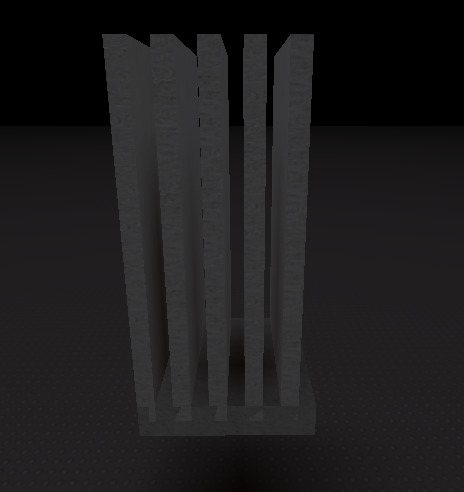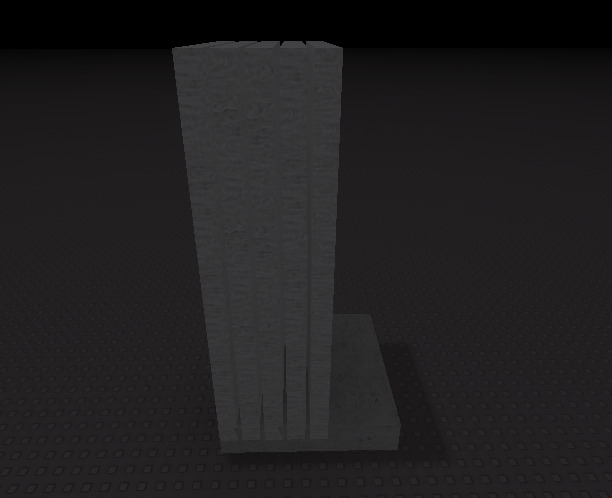# What's the correct ratio?

This is what my current model looks like:However I want the pillars to reach all the way to the other side of the floor with even spacing in between.

What would be the ratio I would have to use to center the walls?

Floor dimensions: 10x10
Wall dimensions: 1x20x5

There is one stud in between each pillar currently

Note that I want this to fit with any size later

1 Like

Floor is 10 studs across and walls are 1 stud thick, meaning that if you place two walls on either side of the floor, you will have 8 studs left over to place the remaining 3 walls.

You need to place a wall every 8/5 = 1.6 studs to maintain even spacing.

EDIT: Just kidding, my math is off. You need to place a wall every 10/8 studs, or 1.25 studs.

Also, this belongs in #help-and-feedback:building-support, as it’s related to modeling. Just a heads up for future referenceHere’s a math equation you can do to calculate this.

Pillar Length=
l−(n×(np−1)) / np

• l is the length of the floor.
• n is the number of units in between the pillars.
• np is the number of pillars.

This is for a 2D space but I’m assuming the width of all of the pillars will be set

1.25 studs worked! But when I used it for other sizes, it stopped working.

As for your other note, I apologize for the lack of information. This is part of a script for a dynamic wall generation system. I just simplified it for the devforum post.

This hits close to home, but in my script, it already know the length of the pillar decided by a wall thickness variable. The spacing is the variable that is missing/needs improvement. Is this how I would change the formula for solve for spacing instead?

Pillar spacing = -l(np)(length/np-1)

That’s the catch, the wall thickness can be anything. I’m basically handing a script to another person to edit so it must be ready for anything. This is why I’m trying to find the proper formula as shown in the above post.

It looks like this could work, I’m away from home right now but when I get back I will try all of the given answers.

You don’t take thickness into account, since you offset the first pillar by half its thickness into the floor. If we take the first and last part thickness into account, the equations are the following:

``````local thickness = 1
local amount = 5
local floorWidth = 10

local start = 0+thickness/2
local _end = floorWidth-thickness/2

--base of equation
local spacing = (_end-start)/amount

--basic maths, just for visualization
spacing = (floorWidth-thickness/2-(0+thickness/2))/amount
spacing = (floorWidth-thickness/2-0-thickness/2)/amount
spacing = (floorWidth-thickness/2*2)/amount

--derived equation
spacing = (floorWidth-thickness)/amount

print(spacing) --(10-1)/5 = 9/5 = 1.8
``````

So after placing the first wall, you have to place the next one `1.8` studs after it.

The equation you should use is `(floorWidth-thickness)/amount` where `floorWidth` is the width of the floor below the parts, `thickness` is the part thickness(assuming it’s the same for all parts, else this equation doesn’t account for it) and `amount` is the number of parts to be placed on the floor. It will give you the offset/spacing of the next part compared to the one before it, starting from the part that is `thickness/2` studs inside the floor part.

@TestAccount563344 I’m going to assume you didn’t account for the pillars themselves, which is why the ratio is too small. However, I am the person who asked the question.@NyrionDev The ratio is a bit large (don’t mind the portion in the center)What we know is that the correct ratio is 1.25, but only on a 10 by 10 grid where there are five pillars

In case I am just doing it wrong, here is the positioning line of code:

``````newwall.Position = Vector3.new(corner.Z + ((i2)*walllengthx), corner.Y + modulesize.Y/2, corner.X + (i1 * (9/5--[[this is where I plugged in your ratios]]))) + Vector3.new(newwall.Size.X/2,0 ,newwall.Size.Z/2) -- move to corner
``````

i1 controls which wall out of 5
same goes for i2 but vice versa

What is the multiplication by `i1`? Is it some kind of sign?

Also, I assume the issue is that you add an extra `newwall.Size.Z/2` to the final spacing due to the second `Vector3` you add to the first one. So try modifying that to: `Vector3.new(newwall.Size.X/2, 0, 0)`

My assumption may be correct because `1.8-0.5 = 1.3` which is close to `1.25` and `0.5` is `newwall.Size.Z/2`(because in your post you say the thickness is 1).

Apologies for the late reply, I was trying to get some data and I was kind of busy. This is some data I collected for the correct number to multiply to get the right alignment

10 x 10: 2.25

20 x 20: 4.75

40 x 40: 9.75

We need to find how the numbers are in common.

This is probably related to you adding extra offsets on top of my function output. However, I see a pattern here. When I remove my outputs from your outputs I get the following values:

2.25-1.8 = 0.45
4.75-3.8 = 0.95
9.75-7.8 = 1.95

When I increase the results by 0.05, the numbers become:

0.5, 1, 2

It’s obvious that when you duplicate the start value(for example from 10 to 20 or from 20 to 40) the resulting gap is also duplicated, however, the main issue is the extra offsets being applied.

According to your pattern, I can draw this formula where c + b = a

c = wallcount/20 - 0.05
b = wallcount/5 - 0.2
a = b + c

where wallcount = 15

c = 15/20 - 0.05 = 0.70
b = 15/5 - 0.2 = 2.8
a = 0.70 + 2.8 = 3.5

Now lets implement it…

It works!
Tysm for the help. I wouldn’t have figured it out without you

I added this in case another person comes across this topic and to explain how it works

I wish this topic could be over, but there is still a small issue. Normally, when I have the wallthickness at 1, it works correctly. But now if I change it to be bigger for example this happens:

Notice that it is slightly off at the end. How do I fix this?

Well that was easy nevermind then. I’ll leave the final line of code here for resource purposes.

``````--For x
local multiplicative = (modulesize.Z/20 - thickness * (0.05)) + (modulesize.Z/5 - thickness * (0.2))
newwall.Position = Vector3.new(corner.X + ((i2)*walllengthx), corner.Y + modulesize.Y/2, corner.Z + (i1 * multiplicative)) + Vector3.new(newwall.Size.X/2,0 ,newwall.Size.Z/2) -- move to corner
--For z
local multiplicative = (modulesize.X/20 - thickness * (0.05)) + (modulesize.X/5 - thickness * (0.2)) --0.05,0.2
newwall.Position = Vector3.new(corner.X + (i1)* multiplicative, corner.Y + modulesize.Y/2, corner.Z + ((i2)*walllengthz)) + Vector3.new(newwall.Size.X/2,0 ,newwall.Size.Z/2) --move to corner
``````

This topic was automatically closed 14 days after the last reply. New replies are no longer allowed.